# Jahn-Teller Distortion - Coordination Chemistry Notes | Study Inorganic Chemistry - Chemistry

## Chemistry: Jahn-Teller Distortion - Coordination Chemistry Notes | Study Inorganic Chemistry - Chemistry

The document Jahn-Teller Distortion - Coordination Chemistry Notes | Study Inorganic Chemistry - Chemistry is a part of the Chemistry Course Inorganic Chemistry.
All you need of Chemistry at this link: Chemistry

JAHN-TELLER DISTORTION

A complex will be regular octahedron when the electronic arrangement in t2g and eg orbitals is symmetric (i.e., both t2g and eg orbitals are electronically non-degenerate) because symmetricall y arranged electrons will repel all the six ligands equally. An electronically non-degenerate means there is onl y one possible arrangement of electrons. For example, the d3 configuration in octahedral symmetry is non-degenerate and symmetric. Only one arrangement is possible for three electrons.

dconfiguration → (t2g)3 e0, only one possible arrangement ( electronically non-degenerate)

When t2g and eorbitals are asymmetrically filled (i.e., either t2g or eg orbitals are electronicall y degenerate) the regular octahedral geometry is not the stable but it transforms into a distorted octahedral symmetry. Electronically degenerate means the situation in which an electron can be arranged in more than one orbitals of equivalent energy. For example, in octahedral Cu 2+ the eg3 configuration is electronically degenerate because it has two possible arrangements of electrons i.e.,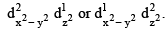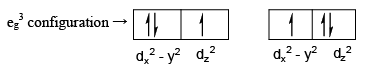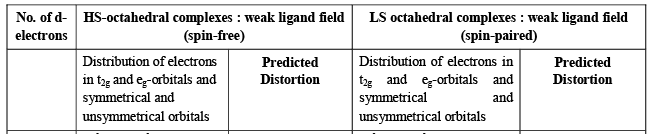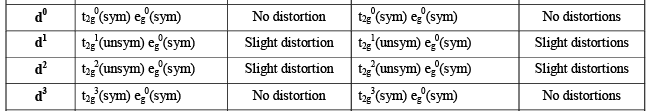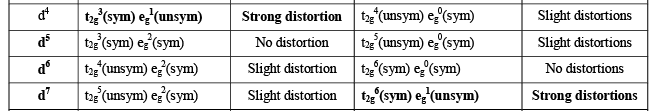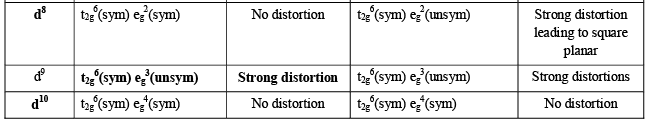Electronically degenerate states for eg3

The eg orbitals point directly towards the ligands, therefore, when e g orbitals are electronicall y degenerate (i.e., unsymmetrically filled), some ligands repelled more than the others. Therefore, there will be significant distortion in octahedral complexes. For example, octahedral complexes of dand high-spin d4 ions are o ften distorted. On the other hand, the t2g orbitals lie in between the ligands; therefore, the electronically degenerate t2g orbitals cause a very small distortion.

t2g (sym) + e(sym) → No distortion
t2g (unsym) → Slight distortion
eg (unsym)  → strong distortion
cases of strong distortion
d4 (High spin), d7 (low spin), d9

The various configurations which cause distortion is given in the following table.
Generally, the distortion that occurs in octahedral complexes is tetragonal distortion. A tetragonal distortion means the two trans ligands on the z-axis in an octahedral complex are moved either towards or away from the metal ion/atom. Motion of trans ligands along z-axis towards the metal atom/ion is called tetragonal compression (z-in distortion) and motion of these ligands away from the metal ion is called the tetragonal elongation (z-out distortion).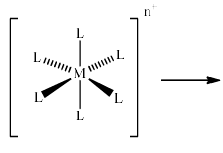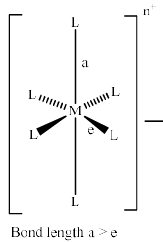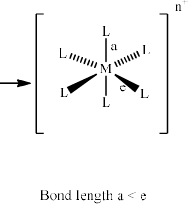The distortion causes by a condition called Jahn-Teller Theorem which states that, “Any non-linear molecular system in a degenerate electronic state will be unstable and will undergo distortion to form a system of lower symmetry and lower energy, thereby removing the degeneracy.” When the t2g set is unequall y occupied then t he distortion occur is very much smaller since t he orbitals are not pointing directly at the ligands. On the other hand when eg orbitals are unsymmetrically filled, the distortion is very strong and z-in or z-out complexes are formed.

z-Out Distortion (Tetragonal Elongation)

In a regular octahedral field, the eg orbitals (i.e., dx2 – y2 and dz2) are degenerate. However, if the eg orbitals are unsymmetrically filled i.e. either dx2 – y2 or dz2 contains one more electron than the other, then the eg orbitals are split. If the dz2 orbitals contain one electron more than the dx2 – y2 orbitals then the ligands approaching along z-axis will be repelled more than the ligands approaching x and y axes. This is due to that electron density in dz2 orbital is greater than that of dx2 – y2 orbital.

(dz2)1 (dx2 – y2 )0 or (dz2)2 (dx2 – y2 )1

Consequently, the ligands along z axis are moved away from the metal cation relative to those on x and y axes which move towards the metal cation. Therefore, the distance between metal cation and the ligands on z-axis becomes larger and the distances between metal cation and ligands on x and y axes becomes shorter. Thus the complex undergoes a tetragonal elongation (z-out distortion).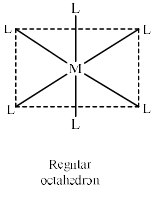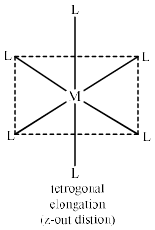The ligands along z axis moved away from the metal cation relative to those on x and y axes which move towards the metal ion. Therefore, the dz2 orbital will experience a decrease in repulsion from the ligands and hence energy of dz2 orbital will decrease i.e. dz2 orbital is stabilised.  On the other hand, the dx2 – y2  experiences a greater repulsion from the ligands and hence the dx2 – y2 will be destabilized. In a similar way, t he energies of orbitals having z component (i.e. dyz and dzx) will decrease and that of dxy will increase in energy and a part of degenerac y of t2g orbitals is removed. Therefore, the overall result is t hat both eg and t2g orbitals split in two levels.  Crystal field splitting in tetragonal elongation (z-out) is shown below: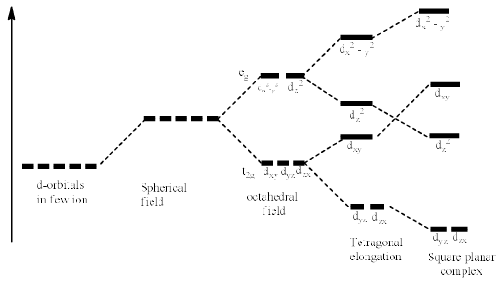Crystal field splitting in tetragonal elongation (z-out distortion)

z-In Distortion (Tetragonal Compression)

If the dx2 – y2 orbital contains one electron more than the dz2 orbitals then the ligands approaching along x and y axes will be repelled more than the ligands approaching along z-axis. This is due to that the electron densit y in dx2 – y2 orbital is greater than that of dz2 orbital.

(dz2)0 (dx2 – y2)1 or (dz2)1 (dx2 – y2)2

Therefore the ligands along x and y axes are moved away from the metal cation relative to those on zaxis which move towards the metal cation. The distance between the metal cation and the ligands is shorter on z-axis because ligand-ligand repulsion is decreased as ligands move away from the metal cation on x and y axes. Therefore, the distance between metal cation and x and y axes becomes larger & become shorter on z-axis. Therefore the complex undergoes tetragonal compression (z-in distortion).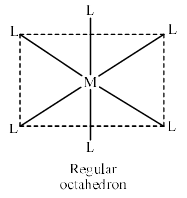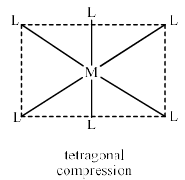Since the ligands on x and y axes are at longer distance from metal cation than that of ligands on z-axis, therefore the dx2 – y2 orbital will decrease in repulsion from the ligands and therefore the energy of  dx2 – y2will decrease. The dzwill experience an increase in repulsion from the ligands resulting in an increase in energy. Likewise, the energies of the orbitals having x and y component (dxy) orbital will decrease and that of having z- component (i.e. dyz and dzx) will increase. The crystal field splitting in tetragonal compression (z-in distortion) is shown below: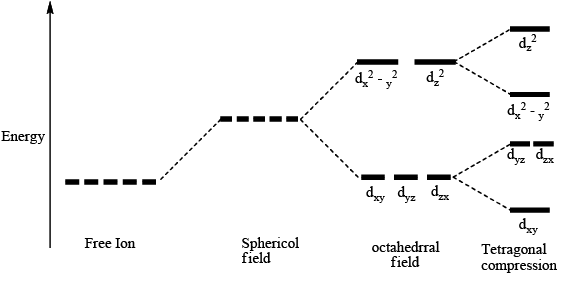Since in tetragonal compression, four ligands on x and y axes repel the high density of electrons in dx2 – yorbital whereas in tetragonal elongation only two ligands on z axis repel the high density of electrons in dz2 orbitals. Therefore, there is more repulsion in tetragonal compression than that of tetragonal elongation. Therefore, tetragonal elongation is more stable than the tetragonal compression and hence tetragonal elongation is known as tetragonal distortion.

Examples of John-Teller Distortion: The significant John-teller distortions are generally observed in high spin d4 (t2g3 eg1), low spin d7 (t2g6 eg1) and d9 (t2g6 eg3) configurations in these configurations eg orbitals are electronically degenerate and points towards the ligands directly. Compounds which undergo strong Jahn-Teller distortion in octahedral field are CrF2 and MnF3 (d4 t2geg1), NaNiO2 (low spin, d7, t2g6 eg1), CuF2, CuCl2, CuBr2 (d9, t2g6 eg3) etc. In all these complexes the eg orbitals are unsymmetrically filled and therefore there are two longer bonds on z-axis and four shorter bonds on x and y axes.

The Jahn-Teller theorem does not predict which type of distortion will take place. There are two types of Jahn Teller distortions:

• Static Jahn-Teller Distortion: Some complexes show tetragonal distortion under all conditions i.e., in solid as well as in solution, either at lower or relatively higher temperatures. This distortion is called static Jahn-Teller distortion. In these complexes the splitting of t2g and eg orbitals is very high so that the thermal energy available will not be sufficient to affect the population of orbitals in a distorted geometry. This type of Jahn-Teller distortion occurs when eg orbitals are electronically degenerate.

Dynamic Jahn-Teller Distortion: In some complexes no distortion is observed at room temperature. In these complexes the thermal energy available at room temperature causes to equalize the population of the tetragonal elongated and tetragonal compressed geometries. But when we lower the temperature the distortion is detected. This is called dynamic Jahn-Teller distortion. For example, the complex [Ti(H2O)6]3+ and [Fe(H2O)6]2+ show dynamic Jahn-Teller distortion. In these complexes both the tetragonal elongation and tetragonal compression exist in equilibrium.
The document Jahn-Teller Distortion - Coordination Chemistry Notes | Study Inorganic Chemistry - Chemistry is a part of the Chemistry Course Inorganic Chemistry.
All you need of Chemistry at this link: ChemistryUse Code STAYHOME200 and get INR 200 additional OFF

## Inorganic Chemistry

49 videos|71 docs|16 tests

Track your progress, build streaks, highlight & save important lessons and more!

,

,

,

,

,

,

,

,

,

,

,

,

,

,

,

,

,

,

,

,

,

;